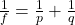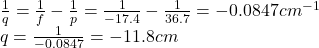## A 3.39 cm tall object is placed in 36.7 cm in front of a convex mirror. The focal length is 17.4 cm. How far is the image from

Question

A 3.39 cm tall object is placed in 36.7 cm in front of a convex mirror. The focal
length is 17.4 cm. How far is the image from the mirror?

in progress 0
3 months 2021-08-30T01:23:53+00:00 1 Answers 2 views 0

-11.8 cm

Explanation:

The position of the image can be found by using the mirror equation:where:

f is the focal length of the mirror

p is the distance of the object from the mirror

q is the distance of the image from the mirror

In this problem, we have:

f = -17.4 cm is the focal length of the mirror (negative for a convex mirror)

p = 36.7 cm is the distance of the object from the mirror

By solving for q, we can find the position of the image from the mirror:And the negative sign indicates that the image is virtual (on the opposite side of the mirror).